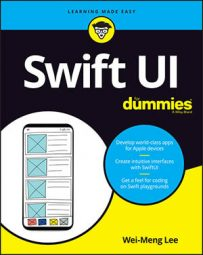##### SwiftUI For DummiesSwift is a type-safe language, which means that the programming language makes it clear to you the types of values your code is working with. The following article discusses how to declare constants and variables and how to work with strings and comments when programming with Swift.

## Swift constants

In Swift, you create a constant using the let keyword:
```let radius = 3.45             // Double
let numOfColumns = 5          // Int
let myName = "Wei-Meng Lee"   // String```
Notice that there is no need to specify the data type — the data types are inferred automatically.

If you want to declare the type of constant, you can do so using the colon operator (:) followed by the data type, as shown here:

`let diameter<strong>:Double</strong> = 8`

After a constant is created, you can no longer change its value. Always use a let when you need to store values that do not change.

## Swift variables

To declare a variable, you use the var keyword:
```var myAge = 25
var circumference = 2 * 3.14 * radius```
After a variable is created, you can change its value. In Swift, values are never implicitly converted to another type. For example, suppose you’re trying to concatenate a string and the value of a variable. In the following example, you need to explicitly use the String() function to convert the value of myAge to a string value before concatenating it with another string:
`var strMyAge = "My age is " + String(myAge)`

To get the text representation of a value (constant or variable), you can also use the description property, like this: myAge.description.

## Swift strings

One of the common tasks in programming is inserting values of variables into a string. In Swift, you use string interpolation and it has the following format:
`"Your string literal <strong>\(</strong><em>variable_name</em><strong>)</strong>"`
The following statement shows an example:
```let firstName = "Wei-Meng"
let lastName = "Lee"
var strName = "My name is \(firstName) \(lastName)"```
You can also use this method to include a Double value in your string (or even perform mathematical operations or function calls):
`var strResult = "The circumference is \(circumference)"`

In Swift, as in most programming languages, you insert comments into your code using two forward slashes (//):
`// this is another comment`
If you have several lines of comments, it’s better to use the /* and */ combination to denote a block of statements as comments:
```/*
this is a comment
this is another comment
*/```